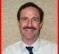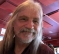## Advice for FM Demodulation TechniquesStarted by 4 years ago13 replieslatest reply 4 years ago718 views

I'm looking for advice on the best way to perform FM Demodulation.

Most methods I've found work with baseband FM signals.

I have a quadrature FM signal that is modulated on a low frequency IF carrier and I need to recover the audio signal.

Any suggestions will be appreciated.

[ - ]So you "have a quadrature FM signal that is modulated on a low frequency IF" which sounds to me that you have both \$i[n]\$ and \$q[n]\$ of a complex signal that has only positive frequency components, correct?

$$s[n] \ = \ i[n] \ + \ j \, q[n]$$

and you want the instantaneous frequency of $s[n]$, correct?  That instantaneous frequency will be biased by the carrier frequency $f_\mathrm{c}$, correct?  This constant term can be later removed with a DC-blocking filter.

So you want to represent your signal in polar form:

\begin{align} s[n] \ &= \ i[n] \ + \ j \, q[n] \\ &= r[n] e*{j \phi[n]} \\ \end{align}

and you want to compute from $s[n]$ how rapidly $\phi[n]$ advances since the derivative of phase is instantaneous frequency, right?

Stephane, I thought we could do math here, but I can't figure out how to do it.  (I know LaTeX math markup.)

[ - ]Thanks for the response.

I thought I had performed what you described but the results weren't as expected.

In MATLAB I generated a 1000 Hz sine wave and modulated a 12500 Hz carrier (with a sample rate of 75000 sps and 750 samples).  I performed a Hilbert transform to end up with complex samples.

Then I took that result and did the following:

I calculated atan(imag(fm)/real(fm)) for all samples.

Then I convoluted that with [1,0,-1].

The result looks kind of like my original sine wave amplitude modulated.

What am I missing?

Greg

[ - ]Firstly did your signal of 1000 Hz have alternating symbols (+/-).

Secondly why are you filtering with that [1,0,-1].

If you are using atan you don't need it.

Thirdly you need to differentiate the atan result to get the symbols back

[ - ]Thank you for the reply, Kaz.

All signals are AC coupled, evenly spaced around zero.

I got that [1,0,-1] filter from Rick Lyon's FM demod paper as a method of performing differentiation.

Greg

[ - ]Hi Greg,

I didn't mean AC issue but by +/- symbols I meant you need two frequencies in your (s) signal each running for a symbol period, preferably continuous in phase as they change from one symbol to next then you apply the demod algorithm.

Kaz

[ - ]Kaz,

I'm not sure what you mean about +/-.

Are you describing the I and Q components of the original signal?

Greg

[ - ]Here is a post by Rick Lyons on FM demodulation.  You can also refer to section 13.22 of his book Understanding Digital Signal Processing (3rd ed.)

https://www.embedded.com/dsp-tricks-frequency-demodulation-algorithms/

One thing to keep in mind:  The noise output of an FM demod goes as f^2.  Normally, you should have a good LPF on the output that cuts off just above the signal band to prevent SNR degradation.

Neil

[ - ]Thanks, Neil.

I read Rick's article before starting this task.  I'm following his figure 13.60.  Modeling in MATLAB with a sine wave I get a result that sort of resembles an amplitude modulated sine wave, but not exactly.

Thanks for the heads up about the noise but the MATLAB model is noise free.

Please see my modeling description above.  What am I missing?

Greg

[ - ]Greg,

Make sure to use atan2(Q,I), and not atan(Q/I).

Another option is to use the block diagram in Figure 13-61 (b).

Neil

[ - ]Post deleted by author

[ - ]Hi

In my SDR receiver, G8JCFSDR, I use the attached code to demodulate FM. It's written in VB so it should be really simple to understand. The vector arithmetic functions use functions from the Intel Signal Processing Library, ISPL, and should be self-explanatory.

Hope this helps

Peter

FMDemodulation.bas

[ - ]Thanks, Peter.

I'm interested.

Nothing was attached.

Can you send it to n9gl@comcast.net?

Thanks again.

Greg

[ - ]Greg

Sent to your email, but just in case your email provider blocks the file, I've pasted the content into this posting itself.

If U haven't got VB, then VSCode does a pretty good job of displaying VB source code.

Peter

'

' Vfm = (I*dQ/dt - Q*dI/dt)/(I^2 + Q^2)

' Demodn={Qn*In-1-In*Qn-1}/{In^2+Qn^2}

'

' In    Real() Array of Real Time Domain Samples

' In    Imag() Array of Imaginary Time Domain Samples

' In    SamplesPerCallback Number of Samples in the Time Domain

' Out   SamplesOut() Array of FM Demodulated Time Domain Samples

'

'

Private Sub DemodulateFM_Phase(ByRef Real() As Single, ByRef Imag() As Single, ByRef SamplesOut() As Single, SamplesPerCallback as Long)

Static J As Long

'Holds the phase of the last sample so that when next we are called

'we will be able to calculate the phase change

Static LastPhase As Single

'Magnitude

Static Mag() As Single

ReDim Preserve Mag(SamplesPerCallback * 2)

'Phase

Static Phase() As Single

ReDim Preserve Phase(SamplesPerCallback * 2)

'Get the Magnitude and Phase angle - this is where ArcTan is used

CartToPolar_S_2 Real(0), Imag(0), Mag(0), Phase(0), SamplesPerCallback

'Find the phase angle changes between each sample

'Special handling for first sample in this new sample block

SamplesOut(0) = (Phase(0) - (LastPhase))

'Save the phase of the last sample in this new sample block

'so that we can use it next time

LastPhase = Phase(SamplesPerCallback - 1)

'Now calculate the phase difference for each sample from the previous sample

For J = 1 To SamplesPerCallback - 1

SamplesOut(J) = (Phase(J) - Phase(J - 1))

Next J

'Take the absolute value of the deltas

Abs_S_1 SamplesOut(0), SamplesPerCallback

'Scale the output a little

Mpy_S_1 256, SamplesOut(0), SamplesPerCallback

'Filter the AF output

AFFilter.Filter_FFT_S_1 SamplesOut()

End Sub